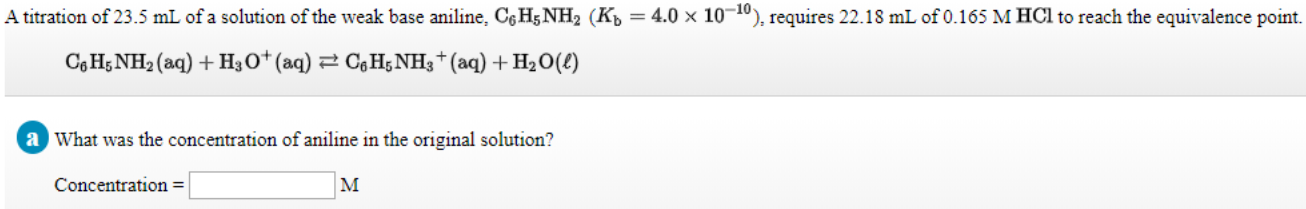# A titration of 23.5 mL of a solution of the weak base aniline, C6H5NH2 (Kb = 4.0 x 10^-10), requires 22.18 mL of 0.165 M HCl to reach the equivalence point. C6H5NH2(aq) + H3O^+(aq) ⇌ C6H5NH3^+(aq) + H2O(ℓ) What was the concentration of aniline in the original solution?